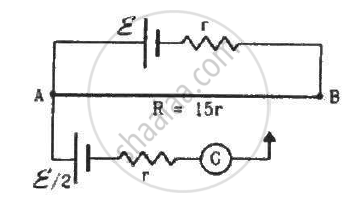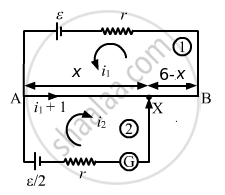Advertisement Remove all ads

# Consider the Potentiometer Circuit as Arranged in the Figure. the Potentiometer Wire is 600 Cm Long. - Physics

Sum

Consider the potentiometer circuit as arranged in the figure. The potentiometer wire is 600 cm long. (a) At what distance from the point A should the  jockey touch the wire to get zero deflection in the galvanometer? (b) If the jockey touches the wire at a distance of 560 cm from A, what will be the current in the galvanometer?Advertisement Remove all ads

#### SolutionLet X be the null point on the wire at a distance x cm from point A, as shown.

Given:-

Total resistance of the wire AB = 15r

Resistance per unit cm = $\frac{15r}{600}$

Resistance of x cm of the wire = $\frac{15rx}{600}$

Resistance of (600 - x ) cm of the wire = $\frac{15r\left( 600 - x \right)}{600}$

(a) Applying KVL in loop 1, we get:-

$\left( i_1 + i_2 \right)\frac{15}{600}rx + \frac{15}{600}r\left( 600 - x \right) i_1 + i_1 r = \epsilon...........(1)$

Applying KVL in loop 2, we get:-

$i_2 r + \frac{15}{600}rx \left( i_1 + i_2 \right) = \frac{\epsilon}{2}............(2)$

For zero deflection in the galvanometer, i2 = 0. From equation (2),

$\frac{15}{600}rx\left( i_1 \right) = \frac{\epsilon}{2}$

$\Rightarrow i_1 = \frac{20\epsilon}{rx}$

Substituting the values of i1 and i2 in equation (1), we get:-

x = 320 cm

(b) Putting x = 560 cm and solving equations (1) and (2), we get:-

$i_2 = \frac{3\epsilon}{22r}$

Is there an error in this question or solution?
Advertisement Remove all ads

#### APPEARS IN

HC Verma Class 11, Class 12 Concepts of Physics Vol. 2
Chapter 10 Electric Current in Conductors
Q 56 | Page 202
Advertisement Remove all ads

#### Video TutorialsVIEW ALL 

Advertisement Remove all ads
Share
Notifications

View all notifications

Forgot password?